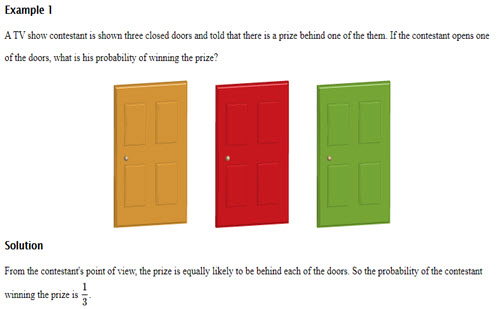Go to website

# Probability

This resource guides and supports the explicit teaching of probability, through discussing the meaning of probability terminology; distinguishing between likely outcomes and outcomes that are not equally likely; and expressing probabilities as decimals, fractions and percentages. Accompanying worked examples and solutions are provided. Student can access the resources to clarify their understanding of probability and to work through sample questions.

Year level(s) Year 7
Audience Teacher, Student
Purpose Content knowledge, Teaching resource, Teaching strategies
Teaching strategies and pedagogical approaches Collaborative learning, Explicit teaching, Worked examples
Keywords probability, likely, unlikely outcome, chosen randomly, sample space, favourable outcomes, trial, experiments and events, chosen randomly, explicit teaching

## Curriculum alignment

Curriculum connections Critical and creative thinking, Numeracy, English
Strand and focus Probability, Statistics, Apply understanding, Build understanding
Topics Chance and probability, Decimals, Fractions, Percentage
AC: Mathematics content descriptions
ACMSP167 Construct sample spaces for single-step experiments with equally likely outcomes
ACMSP168 Assign probabilities to the outcomes of events and determine probabilities for events
National numeracy learning progression Understanding chance - UnC5